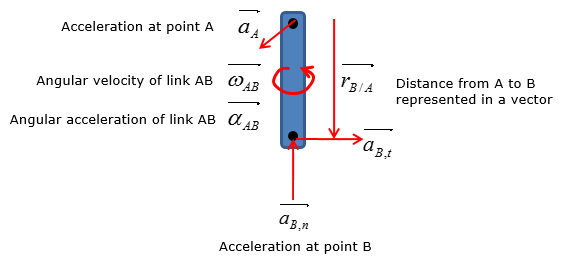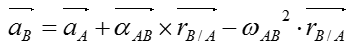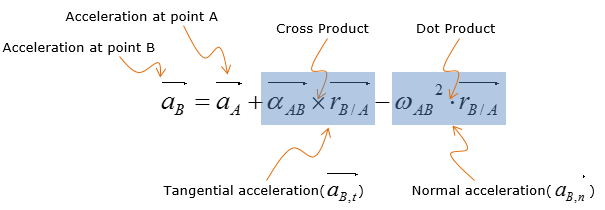Mechanical Engineering - Linkage System                                 Home : www.sharetechnote.com Linkage System : Relative Motion Analysis : Acceleration   All of linkage system problems involve relative-motion analysis because the motion of a link is affected directly by the motion of its adjacent link. You must be comfortable with setting up vectors as well as executing vector addition, subtraction, and multiplication by hand. And you must know the magnitude of angular velocity to calculate acceleration of a link at a certain point of interest. Scalar method cannot be used for the acceleration analysis   Let's consider a simple linkage system as shown belowAcceleration of the link at point B is calculated by using the following equation if vector method is to be usedPutting a short description on this equation as follows would help you with understanding this equation. The equation states that the acceleration at point B of the rotating link AB is dependent on the sum of the acceleration at point A and acceleration of point B with respect to point A